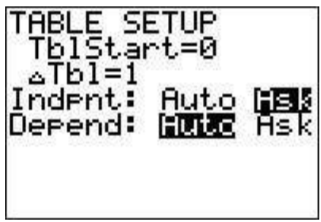# 26.5: A.5- Using the table

$$\newcommand{\vecs}{\overset { \rightharpoonup} {\mathbf{#1}} }$$ $$\newcommand{\vecd}{\overset{-\!-\!\rightharpoonup}{\vphantom{a}\smash {#1}}}$$$$\newcommand{\id}{\mathrm{id}}$$ $$\newcommand{\Span}{\mathrm{span}}$$ $$\newcommand{\kernel}{\mathrm{null}\,}$$ $$\newcommand{\range}{\mathrm{range}\,}$$ $$\newcommand{\RealPart}{\mathrm{Re}}$$ $$\newcommand{\ImaginaryPart}{\mathrm{Im}}$$ $$\newcommand{\Argument}{\mathrm{Arg}}$$ $$\newcommand{\norm}{\| #1 \|}$$ $$\newcommand{\inner}{\langle #1, #2 \rangle}$$ $$\newcommand{\Span}{\mathrm{span}}$$ $$\newcommand{\id}{\mathrm{id}}$$ $$\newcommand{\Span}{\mathrm{span}}$$ $$\newcommand{\kernel}{\mathrm{null}\,}$$ $$\newcommand{\range}{\mathrm{range}\,}$$ $$\newcommand{\RealPart}{\mathrm{Re}}$$ $$\newcommand{\ImaginaryPart}{\mathrm{Im}}$$ $$\newcommand{\Argument}{\mathrm{Arg}}$$ $$\newcommand{\norm}{\| #1 \|}$$ $$\newcommand{\inner}{\langle #1, #2 \rangle}$$ $$\newcommand{\Span}{\mathrm{span}}$$$$\newcommand{\AA}{\unicode[.8,0]{x212B}}$$

The table ($$\boxed{\text{2nd}}$$$$\boxed{\text{graph}}$$) is useful as it gives a table representation of a function (from the $$\boxed{y=}$$ screen). Make sure there is a function typed in the Y1 postion and look at the table. If you want to know the value of the function for particular values of $$x$$ then we must use the ’TBLSET’ screen. Press $$\boxed{\text{2nd}}$$$$\boxed{\text{window}}$$. Move the cursor to the ’Indpnt’ line then to the right to highlight ask and press $$\boxed{\text{enter}}$$.Go back to the table $$\boxed{\text{2nd}}$$$$\boxed{\text{graph}}$$. Now your table is empty. Evaluate your function at $$2.5$$ by typing $$2.5$$ and pressing $$\boxed{\text{enter}}$$. You will see the value of the function in Y1 at $$2.5$$ in the table now. You can evaluate at any number of points.You can also evaluate at every half integer starting at $$-1$$ for example by going to the ’TBLSET’ screen ($$\boxed{\text{2nd}}$$$$\boxed{\text{window}}$$) changing ’TblStart’ to $$\boxed{\text{(-)}}$$$$\boxed{\text{1}}$$and $$\Delta$$Tbl$$=.5$$. Also change the ‘Indpnt’ setting to ‘Auto’ again.View the table ($$\boxed{\text{2nd}}$$$$\boxed{\text{graph}}$$) to see what happened.

This page titled 26.5: A.5- Using the table is shared under a CC BY-NC-SA 4.0 license and was authored, remixed, and/or curated by Thomas Tradler and Holly Carley (New York City College of Technology at CUNY Academic Works) via source content that was edited to the style and standards of the LibreTexts platform; a detailed edit history is available upon request.